# Fraction calculator

The calculator performs basic and advanced operations with fractions, expressions with fractions combined with integers, decimals, and mixed numbers. It also shows detailed step-by-step information about the fraction calculation procedure. Solve problems with two, three, or more fractions and numbers in one expression.

## Result:

### (31/4) : 6 = 13/24 ≅ 0.5416667

Spelled result in words is thirteen twenty-fourths.

### How do you solve fractions step by step?

1. Conversion a mixed number 3 1/4 to a improper fraction: 3 1/4 = 3 1/4 = 3 · 4 + 1/4 = 12 + 1/4 = 13/4

To find a new numerator:
a) Multiply the whole number 3 by the denominator 4. Whole number 3 equally 3 * 4/4 = 12/4
b) Add the answer from previous step 12 to the numerator 1. New numerator is 12 + 1 = 13
c) Write a previous answer (new numerator 13) over the denominator 4.

Three and one quarter is thirteen quarters
2. Divide: 13/4 : 6 = 13/4 · 1/6 = 13 · 1/4 · 6 = 13/24
Dividing two fractions is the same as multiplying the first fraction by the reciprocal value of the second fraction. The first sub-step is to find the reciprocal (reverse the numerator and denominator, reciprocal of 6/1 is 1/6) of the second fraction. Next, multiply the two numerators. Then, multiply the two denominators. In the following intermediate step, the fraction result cannot be further simplified by canceling.
In other words - thirteen quarters divided by six = thirteen twenty-fourths.

#### Rules for expressions with fractions:

Fractions - use the slash “/” between the numerator and denominator, i.e., for five-hundredths, enter 5/100. If you are using mixed numbers, be sure to leave a single space between the whole and fraction part.
The slash separates the numerator (number above a fraction line) and denominator (number below).

Mixed numerals (mixed fractions or mixed numbers) write as non-zero integer separated by one space and fraction i.e., 1 2/3 (having the same sign). An example of a negative mixed fraction: -5 1/2.
Because slash is both signs for fraction line and division, we recommended use colon (:) as the operator of division fractions i.e., 1/2 : 3.

Decimals (decimal numbers) enter with a decimal point . and they are automatically converted to fractions - i.e. 1.45.

The colon : and slash / is the symbol of division. Can be used to divide mixed numbers 1 2/3 : 4 3/8 or can be used for write complex fractions i.e. 1/2 : 1/3.
An asterisk * or × is the symbol for multiplication.
Plus + is addition, minus sign - is subtraction and ()[] is mathematical parentheses.
The exponentiation/power symbol is ^ - for example: (7/8-4/5)^2 = (7/8-4/5)2

#### Examples:

subtracting fractions: 2/3 - 1/2
multiplying fractions: 7/8 * 3/9
dividing Fractions: 1/2 : 3/4
exponentiation of fraction: 3/5^3
fractional exponents: 16 ^ 1/2
adding fractions and mixed numbers: 8/5 + 6 2/7
dividing integer and fraction: 5 ÷ 1/2
complex fractions: 5/8 : 2 2/3
decimal to fraction: 0.625
Fraction to Decimal: 1/4
Fraction to Percent: 1/8 %
comparing fractions: 1/4 2/3
multiplying a fraction by a whole number: 6 * 3/4
square root of a fraction: sqrt(1/16)
reducing or simplifying the fraction (simplification) - dividing the numerator and denominator of a fraction by the same non-zero number - equivalent fraction: 4/22
expression with brackets: 1/3 * (1/2 - 3 3/8)
compound fraction: 3/4 of 5/7
fractions multiple: 2/3 of 3/5
divide to find the quotient: 3/5 ÷ 2/3

The calculator follows well-known rules for order of operations. The most common mnemonics for remembering this order of operations are:
PEMDAS - Parentheses, Exponents, Multiplication, Division, Addition, Subtraction.
BEDMAS - Brackets, Exponents, Division, Multiplication, Addition, Subtraction
BODMAS - Brackets, Of or Order, Division, Multiplication, Addition, Subtraction.
GEMDAS - Grouping Symbols - brackets (){}, Exponents, Multiplication, Division, Addition, Subtraction.
Be careful, always do multiplication and division before addition and subtraction. Some operators (+ and -) and (* and /) has the same priority and then must evaluate from left to right.

## Fractions in word problems:

• Fraction of a fractionWhat is two-quarters of twelve?
• Percent calculationCalculate 8% if 44% is 32.
• DayWhat part of the day are 23 hours 22 minutes? Express as a decimal number.
• Scale of the mapDetermine the map's scale, which is the actual distance of 120 km l represented by a segment long 6 cm.
• Candy barsSheldon has 4 candy bars and wants to split them among his five friends. If each person gets the same amount, what part of the candy bar will each friend get? Show your work.
• Container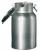How many three-quarters liters jar fill 30 liters container?
• Reciprocal value 2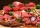Annabelle is making burger patties if each burger patty needs 1/16 kilogram of beef. How many patties can she make with a kilo of beef?
• Bubble bee marblesMark has 100 marbles. Seventeen are bubble bee marbles. What decimal number shows the fraction of marbles that are bumblebees?
• 5 books5 books cost \$28.75. Justin wants to buy 8 similar books. He has \$50. Does he have enough?
• The bird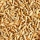The bird feeder holds 1 and 1/2 cups of birdseed. Reagan is using a scoop that holds 1/8 of a cup. How many scoops will she need to fill the bird feeder?
• Fraction unknowns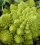Divide of fractions with unknowns: Fraction 1: The quantity x squared plus 6 times x plus 9 over the quantity x minus 1. Fraction 2: the quantity x squared minus 9 over the quantity x squared minus 2 times x plus 1.  Find Fraction 1 over Fraction 2.
• A glass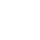A glass holds 3/4 cups. A jug holds 12 cups. How many glasses can be filled?
• 6/24 change6/24 change to a fraction in lowest terms